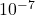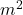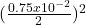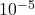## a soleinoid of length 2.8 cm and diamter 0.75 cm is wound with 160 turns per cm. When the current through the solenoid is 0.20 A, what is th

Question

a soleinoid of length 2.8 cm and diamter 0.75 cm is wound with 160 turns per cm. When the current through the solenoid is 0.20 A, what is the magnetic flux through one of the windings of the solenoid

in progress 0
3 weeks 2021-08-30T16:28:52+00:00 1 Answers 0 views 0

1.77 xWb

Explanation:

the magnitude of force given by one of the windings is given by “

Ф = BA = ∪nIA

where B is the magnetic field strength inside the solenoid

A is cross-sectiona area of the windings in= π x= 4.418 x$$m^{2}$$

∪ is the vacuum permeability = 4π xH/m

n i s given by n is number of turns per m = 160 x 100 per m = 16000/m

Ф  = 4  xx 3.142  x 16000 x  0.20 x 4.418 x= 1.77 xWb